Name:    AP Statistics 10 MC Practice

Multiple Choice
Identify the letter of the choice that best completes the statement or answers the question.

1.

You want to compute a 96% confidence interval for a population mean.  Assume that the population standard deviation is known to be 10 and the sample size is 50.  The critical value to be used in this calculation is
 a. 1.960 b. 1.645 c. 1.751 d. 2.054 e. None of the above.

2.

You have measured the systolic blood pressure of a random sample of 25 employees of a company located near you.  A 95% confidence interval for the mean systolic blood pressure for the employees of this company is (122, 138).  Which of the following statements gives a valid interpretation of this interval?
 a. Ninety-five percent of the sample of employees have a systolic blood pressure between 122 and 138. b. Ninety-five percent of the population of employees have a systolic blood pressure between 122 and 138. c. If the procedure were repeated many times, 95% of the resulting confidence intervals would contain the population mean systolic blood pressure. d. The probability that the population mean blood pressure is between 122 and 138 is 0.95. e. If the procedure were repeated many times, 95% of the sample means would be between 122 and 138.

3.

An analyst, using a random sample of n = 500 families, obtained a 90% confidence interval for mean monthly family income for a large population: (\$600, \$800). If the analyst had used a 99% confidence level instead, the confidence interval would be:
 a. Narrower and would involve a larger risk of being incorrect b. Wider and would involve a smaller risk of being incorrect c. Narrower and would involve a smaller risk of being incorrect d. Wider and would involve a larger risk of being incorrect e. Wider but it cannot be determined whether the risk of being incorrect would be larger or smaller

4.

In an opinion poll, 25% of a random sample of 200 people said that they were strongly opposed to having a state lottery.  The standard error of the sample proportion is approximately
 a. 0.03 b. 0.25 c. 0.0094 d. 6.12 e. 0.06

5.

In preparing to use a t procedure, suppose we were not sure if the population was Normal.  In which of the following circumstances would we not be safe using a t procedure?
 a. A stemplot of the data is roughly bell-shaped. b. A histogram of the data shows moderate skewness. c. A stemplot of the data has a large outlier. d. The sample standard deviation is large. e. The t procedures are robust, so it is always safe.

6.

In a poll, (a) some people refused to answer questions, (b) people without telephones could not be in the sample, and (c) some people never answered the phone in several calls.  Which of these sources is included in the ±2% margin of error announced for the poll?
 a. Only source (a). b. Only source (b). c. Only source (c). d. All three sources of error. e. None of these sources of error.

7.

You want to compute a 90% confidence interval for the mean of a population with unknown population standard deviation.  The sample size is 30.  The value of t* you would use for this interval is
 a. 1.96 b. 1.645 c. 1.699 d. 0.9 e. 1.311

8.

The college newspaper of a large Midwestern university periodically conducts a survey of students on campus to determine the attitude on campus concerning issues of interest.  Pictures of the students interviewed along with quotes of their responses are printed in the paper.  Students are interviewed by a reporter “roaming” the campus selecting students to interview “haphazardly.”  On a particular day the reporter interviews five students and asks them if they feel there is adequate student parking on campus.  Four of the students say, “no.” Which of the following conditions for inference about a proportion using a confidence interval are violated in this example?
 a. The data are an SRS from the population of interest. b. The population is at least ten times as large as the sample. c.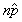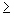10 and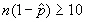. d. We are interested in inference about a proportion. e. More than one condition is violated.

9.

To assess the accuracy of a laboratory scale, a standard weight that is known to weigh 1 gram is repeatedly weighed a total of n times and the mean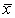of the weighings is computed. Suppose the scale readings are Normally distributed with unknown mean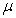and standard deviation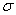= 0.01 g. How large should n be so that a 95% confidence interval forhas a margin of error of ± 0.0001?
 a. 100 b. 196 c. 27,061 d. 10,000 e. 38,416

10.

Many television viewers express doubts about the validity of certain commercials.  In an attempt to answer their critics, the Timex Corporation wishes to estimate the proportion of consumers who believe what is shown in Timex television commercials.  Let p represent the true proportion of consumers who believe what is shown in Timex television commercials.  If Timex has no prior information regarding the true value of p, how many consumers should be included in their sample so that they will be 95% confident that their estimate is within 0.03 of the true value of p?
 a. 202 b. 203 c. 1067 d. 1068 e. 1165